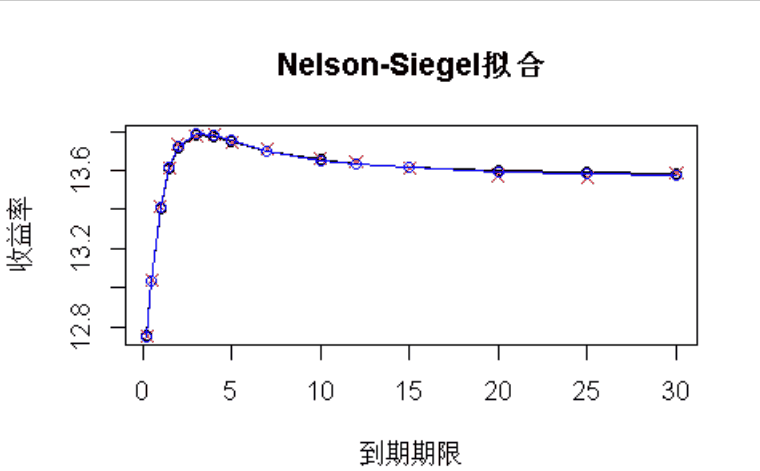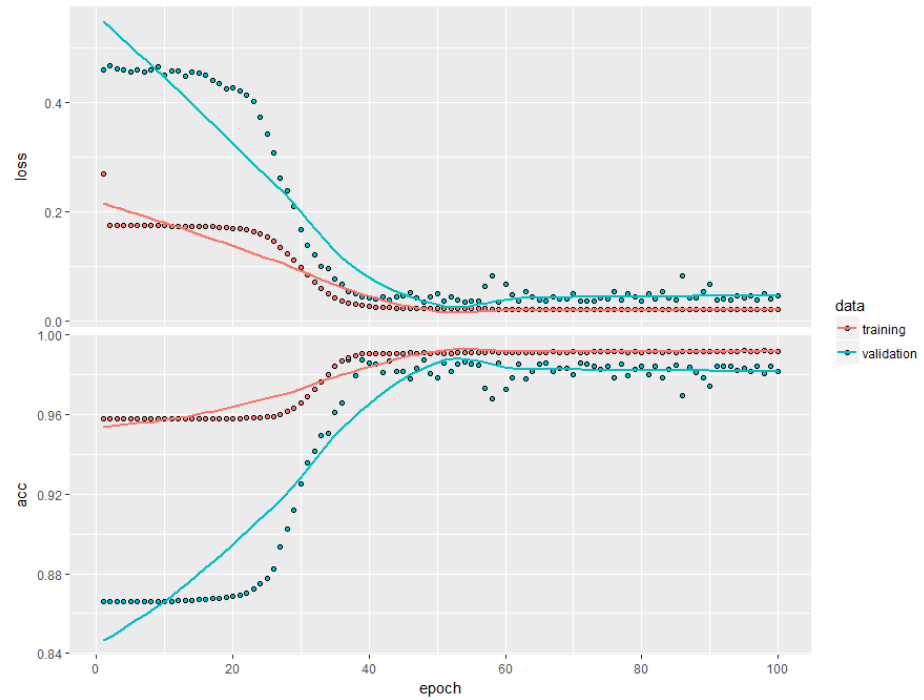# R语言用神经网络改进Nelson-Siegel模型拟合收益率曲线分析

Nelson-Siegel- [Svensson]模型是拟合收益曲线的常用方法。它的优点是其参数的经济可解释性，被银行广泛使用

`````` for(j in 1:N_SIMULATIONS)

{

npo = c(newYields, oldYields)

plot(MATURITY_BASES, oldYields, ylim=c(min(npo), max(npo)))

lines(MATURITY_BASES, oldYields)

points(MATURITY_BASES, newYields, col="red", pch=4)

points(newMATs, newNsYields, col="blue")

lines(newMATs, newNsYields, col="blue") ````````maxDistanceArray[j] = max( abs(oldYieldsArray[j,] - newNsYieldsArray[j,]) )``

_maxDistanceArray_的概率密度   如下所示：`````` idx = intersect(intersect(which(b0 < q_b0), which(b1 < q_b1)), which(b2 < q_b2))

par(mfrow=c(3,3))

plot(density(log(b0)))

plot(density(log(b1)))

plot(density(log(b2)))

plot(density(log(b0[idx])))

plot(density(log(b1[idx])))

plot(density(log(b2[idx])))

plot(density(b0[idx]))

plot(density(b1[idx]))

plot(density(b2[idx]))

b0 = b0-mean(b0)

b1 = b1-mean(b1)

b2 = b2-mean(b2)

#训练神经网络

X = cbind(b0, b1, b2)

Y = array(0, dim=(N_SIMULATIONS-1))

Y[idx] = 1``````

# 用R语言用Nelson Siegel和线性插值模型对债券价格和收益率建模

``````SPLT = 0.8

library(keras)

b = floor(SPLT*(N_SIMULATIONS-1))

plot(history)

model %>% evaluate(x_test, y_test)``````# 神经网络不仅在训练集而且在验证集上都提供了高精度。

Kaizong Ye拓端研究室（TRL）的研究员。

​非常感谢您阅读本文，如需帮助请联系我们！QQ在线咨询

15121130882

0571-63341498

## 关注有关新文章的微信公众号

This will close in 0 seconds